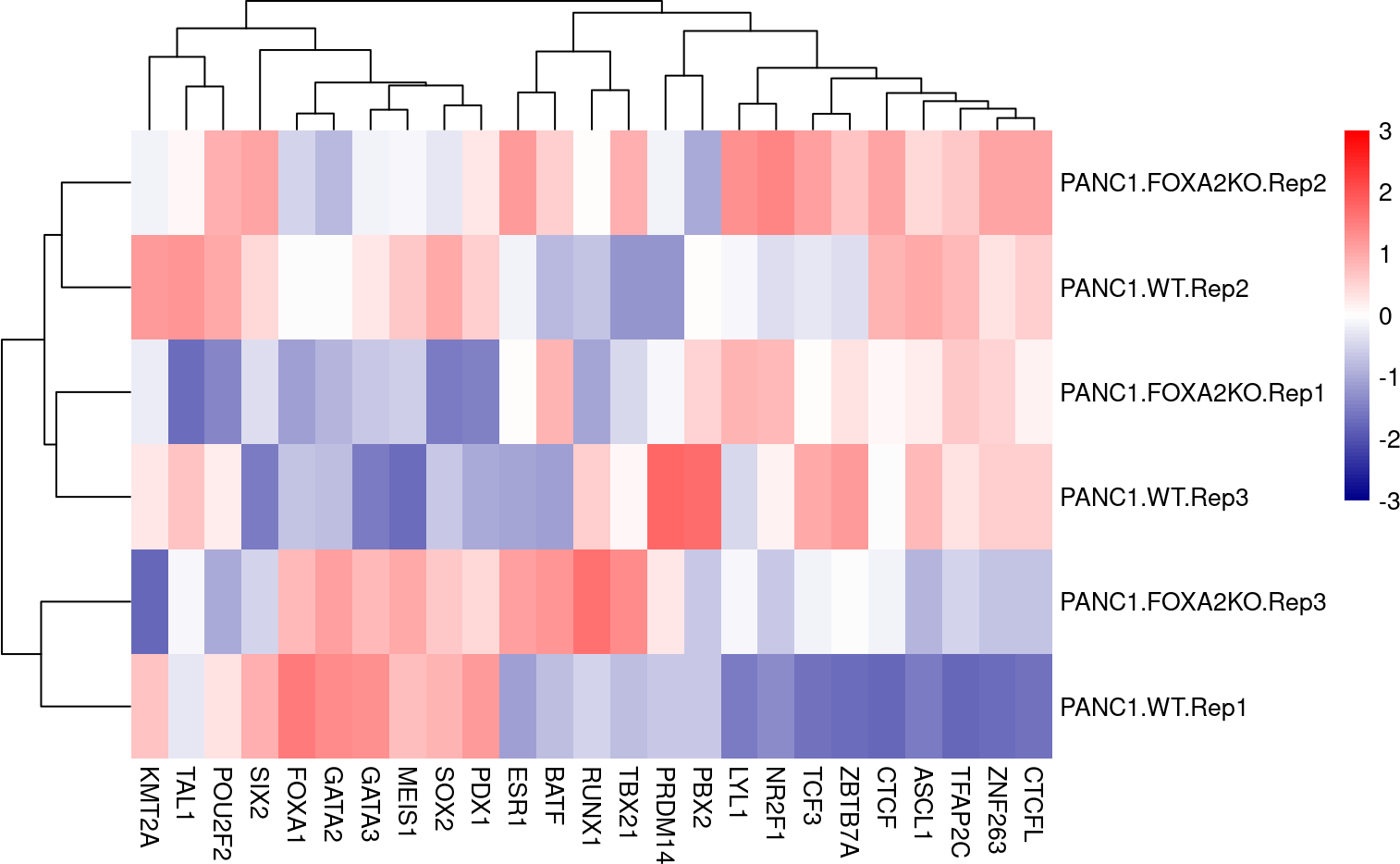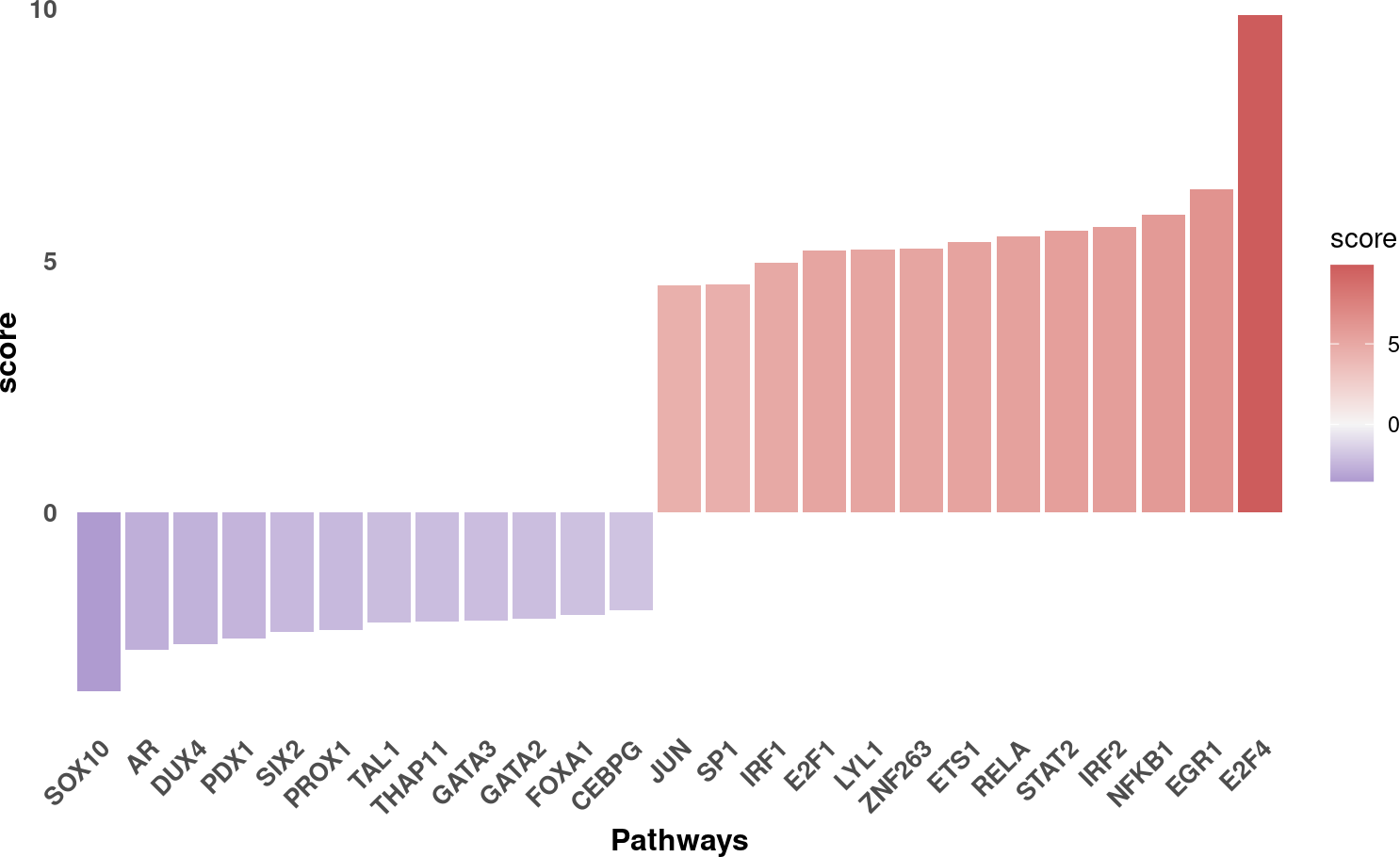Bulk RNA-seq yield many molecular readouts that are hard to interpret by themselves. One way of summarizing this information is by inferring transcription factor (TF) activities from prior knowledge.

In this notebook we showcase how to use `decoupleR` for transcription factor activity inference with a bulk RNA-seq data-set where the transcription factor FOXA2 was knocked out in pancreatic cancer cell lines.

The data consists of 3 Wild Type (WT) samples and 3 Knock Outs (KO). They are freely available in GEO.

First, we need to load the relevant packages:

``````## We load the required packages
library(decoupleR)
library(dplyr)
library(tibble)
library(tidyr)
library(ggplot2)
library(pheatmap)
library(ggrepel)``````

Here we used an already processed bulk RNA-seq data-set. We provide the normalized log-transformed counts, the experimental design meta-data and the Differential Expressed Genes (DEGs) obtained using `limma`. We can open the data like this:

``````inputs_dir <- system.file("extdata", package = "decoupleR")

From `data` we can extract the mentioned information. Here we see the normalized log-transformed counts:

``````# Remove NAs and set row names
counts <- data\$counts %>%
dplyr::mutate_if(~ any(is.na(.x)), ~ if_else(is.na(.x),0,.x)) %>%
column_to_rownames(var = "gene") %>%
as.matrix()
#>          PANC1.WT.Rep1 PANC1.WT.Rep2 PANC1.WT.Rep3 PANC1.FOXA2KO.Rep1 PANC1.FOXA2KO.Rep2 PANC1.FOXA2KO.Rep3
#> NOC2L        10.052588     11.949123     12.057774          12.312291          12.139918          11.494205
#> PLEKHN1       7.535115      8.125993      8.714880           8.048196           8.290154           8.621239
#> PERM1         6.281242      6.424582      6.589668           6.293285           6.486136           6.775344
#> ISG15        10.938252     11.469081     11.425415          11.549986          11.371464          11.178157
#> AGRN          6.956335      7.196108      7.522550           7.061549           7.485534           7.071555
#> C1orf159      9.546224      9.788721      9.794589           9.850830           9.988069           9.965357``````

The design meta-data:

``````design <- data\$design
design
#> # A tibble: 6 × 2
#>   sample             condition
#>   <chr>              <chr>
#> 1 PANC1.WT.Rep1      PANC1.WT
#> 2 PANC1.WT.Rep2      PANC1.WT
#> 3 PANC1.WT.Rep3      PANC1.WT
#> 4 PANC1.FOXA2KO.Rep1 PANC1.FOXA2KO
#> 5 PANC1.FOXA2KO.Rep2 PANC1.FOXA2KO
#> 6 PANC1.FOXA2KO.Rep3 PANC1.FOXA2KO``````

And the results of `limma`, of which we are interested in extracting the obtained t-value and p-value from the contrast:

``````# Extract t-values per gene
deg <- data\$limma_ttop %>%
select(ID, logFC, t, P.Value) %>%
filter(!is.na(t)) %>%
column_to_rownames(var = "ID") %>%
as.matrix()
#>             logFC          t      P.Value
#> RHBDL2  -1.823940 -12.810588 3.030276e-06
#> PLEKHH2 -1.568830 -10.794453 9.932046e-06
#> HEG1    -1.725806  -9.788112 1.939734e-05
#> CLU     -1.786200  -9.761618 1.975813e-05
#> FHL1     2.087082   8.950191 3.552199e-05
#> RBP4    -1.728960  -8.529074 4.904579e-05``````

# 3 DoRothEA network

DoRothEA is a comprehensive resource containing a curated collection of TFs and their transcriptional targets. Since these regulons were gathered from different types of evidence, interactions in DoRothEA are classified in different confidence levels, ranging from A (highest confidence) to D (lowest confidence). Moreover, each interaction is weighted by its confidence level and the sign of its mode of regulation (activation or inhibition).

For this example we will use the human version (mouse is also available) and we will use the confidence levels ABC. We can use `decoupleR` to retrieve it from `OmniPath`:

``````net <- get_dorothea(organism='human', levels=c('A', 'B', 'C'))
net
#> # A tibble: 32,277 × 4
#>    source confidence target     mor
#>    <chr>  <chr>      <chr>    <dbl>
#>  1 ADNP   C          ATF7IP   0.333
#>  2 ADNP   C          DYRK1A   0.333
#>  3 ADNP   C          TLK1     0.333
#>  4 ADNP   C          ZMYM4    0.333
#>  5 AHR    C          ARHGAP15 0.333
#>  6 AHR    C          ARID5B   0.333
#>  7 AHR    B          ASAP1    0.5
#>  8 AHR    C          CREB5    0.333
#>  9 AHR    C          CTNNA1   0.333
#> 10 AHR    C          CTNNA2   0.333
#> # … with 32,267 more rows``````

# 4 Activity inference with Weighted Mean

To infer activities we will run the Weighted Mean method (`wmean`). It infers regulator activities by first multiplying each target feature by its associated weight which then are summed to an enrichment score `wmean`. Furthermore, permutations of random target features can be performed to obtain a null distribution that can be used to compute a z-score `norm_wmean`, or a corrected estimate `corr_wmean` by multiplying `wmean` by the minus log10 of the obtained empirical p-value.

In this example we use `wmean` but we could have used any other. To see what methods are available use `show_methods()`.

To run `decoupleR` methods, we need an input matrix (`mat`), an input prior knowledge network/resource (`net`), and the name of the columns of net that we want to use.

``````# Run wmean
sample_acts <- run_wmean(mat=counts, net=net, .source='source', .target='target',
.mor='mor', times = 100, minsize = 5)
sample_acts
#> # A tibble: 5,040 × 5
#>    statistic  source condition           score p_value
#>    <chr>      <chr>  <chr>               <dbl>   <dbl>
#>  1 corr_wmean AHR    PANC1.FOXA2KO.Rep1  1.90     0.62
#>  2 corr_wmean AHR    PANC1.FOXA2KO.Rep2  2.25     0.58
#>  3 corr_wmean AHR    PANC1.FOXA2KO.Rep3  2.59     0.52
#>  4 corr_wmean AHR    PANC1.WT.Rep1       3.24     0.44
#>  5 corr_wmean AHR    PANC1.WT.Rep2       0.697    0.84
#>  6 corr_wmean AHR    PANC1.WT.Rep3       1.78     0.64
#>  7 corr_wmean AR     PANC1.FOXA2KO.Rep1  6.29     0.2
#>  8 corr_wmean AR     PANC1.FOXA2KO.Rep2  3.54     0.4
#>  9 corr_wmean AR     PANC1.FOXA2KO.Rep3  4.41     0.32
#> 10 corr_wmean AR     PANC1.WT.Rep1      15.5      0.02
#> # … with 5,030 more rows``````

# 5 Visualization

From the obtained results, we will select the `norm_wmean` activities and we will observe the most variable activities across samples in a heat-map:

``````n_tfs <- 25

# Transform to wide matrix
sample_acts_mat <- sample_acts %>%
filter(statistic == 'norm_wmean') %>%
pivot_wider(id_cols = 'condition', names_from = 'source',
values_from = 'score') %>%
column_to_rownames('condition') %>%
as.matrix()

# Get top tfs with more variable means across clusters
tfs <- sample_acts %>%
group_by(source) %>%
summarise(std = sd(score)) %>%
arrange(-abs(std)) %>%
pull(source)
sample_acts_mat <- sample_acts_mat[,tfs]

# Scale per sample
sample_acts_mat <- scale(sample_acts_mat)

# Choose color palette
palette_length = 100
my_color = colorRampPalette(c("Darkblue", "white","red"))(palette_length)

my_breaks <- c(seq(-3, 0, length.out=ceiling(palette_length/2) + 1),
seq(0.05, 3, length.out=floor(palette_length/2)))

# Plot
pheatmap(sample_acts_mat, border_color = NA, color=my_color, breaks = my_breaks) ``````We can observe that WT samples have higher activities for PDX1 and SIX2 than KO. On the other hand, KO show higher activities for LYL1 and ZNF263.

We can also infer pathway activities from the t-values of the DEGs between KO and WT:

``````# Run wmean
contrast_acts <- run_wmean(mat=deg[, 't', drop=FALSE], net=net, .source='source', .target='target',
.mor='mor', times = 100, minsize = 5)
contrast_acts
#> # A tibble: 840 × 5
#>    statistic  source condition   score p_value
#>    <chr>      <chr>  <chr>       <dbl>   <dbl>
#>  1 corr_wmean AHR    t          0.0167    0.7
#>  2 corr_wmean AR     t         -0.508     0.02
#>  3 corr_wmean ARID2  t          0.0129    0.74
#>  4 corr_wmean ARID3A t          0.415     0.18
#>  5 corr_wmean ARNT   t          0.0117    0.74
#>  6 corr_wmean ARNTL  t          0.0369    0.6
#>  7 corr_wmean ASCL1  t          0.0222    0.78
#>  8 corr_wmean ATF1   t          0.0563    0.52
#>  9 corr_wmean ATF2   t          0.283     0.18
#> 10 corr_wmean ATF3   t          0.0262    0.74
#> # … with 830 more rows``````

We select the `norm_wmean` activities and then we show the changes in activity between KO and WT:

``````# Filter norm_wmean
f_contrast_acts <- contrast_acts %>%
filter(statistic == 'norm_wmean') %>%
mutate(rnk = NA)

# Filter top TFs in both signs
msk <- f_contrast_acts\$score > 0
f_contrast_acts[msk, 'rnk'] <- rank(-f_contrast_acts[msk, 'score'])
f_contrast_acts[!msk, 'rnk'] <- rank(-abs(f_contrast_acts[!msk, 'score']))
tfs <- f_contrast_acts %>%
arrange(rnk) %>%
pull(source)
f_contrast_acts <- f_contrast_acts %>%
filter(source %in% tfs)

# Plot
ggplot(f_contrast_acts, aes(x = reorder(source, score), y = score)) +
geom_bar(aes(fill = score), stat = "identity") +
scale_fill_gradient2(low = "darkblue", high = "indianred",
mid = "whitesmoke", midpoint = 0) +
theme_minimal() +
theme(axis.title = element_text(face = "bold", size = 12),
axis.text.x =
element_text(angle = 45, hjust = 1, size =10, face= "bold"),
axis.text.y = element_text(size =10, face= "bold"),
panel.grid.major = element_blank(),
panel.grid.minor = element_blank()) +
xlab("Pathways")``````As observed before, the pathways PDX1 and SIX2 are deactivated in KO when compared to WT, while LYL1 and ZNF263 seem to be activated.

We can further visualize the most differential target genes in each TF along their p-values to interpret the results. For example, let’s see the genes that are belong to FOXA2:

``````tf <- 'FOXA2'

df <- net %>%
filter(source == tf) %>%
arrange(target) %>%
mutate(ID = target, color = "3") %>%
column_to_rownames('target')

inter <- sort(intersect(rownames(deg),rownames(df)))
df <- df[inter, ]
df[,c('logfc', 't_value', 'p_value')] <- deg[inter, ]
df <- df %>%
mutate(color = if_else(mor > 0 & t_value > 0, '1', color)) %>%
mutate(color = if_else(mor > 0 & t_value < 0, '2', color)) %>%
mutate(color = if_else(mor < 0 & t_value > 0, '2', color)) %>%
mutate(color = if_else(mor < 0 & t_value < 0, '1', color))

ggplot(df, aes(x = logfc, y = -log10(p_value), color = color, size=abs(mor))) +
geom_point() +
scale_colour_manual(values = c("red","royalblue3","grey")) +
geom_label_repel(aes(label = ID, size=1)) +
theme_minimal() +
theme(legend.position = "none") +
geom_vline(xintercept = 0, linetype = 'dotted') +
geom_hline(yintercept = 0, linetype = 'dotted') +
ggtitle(tf)``````